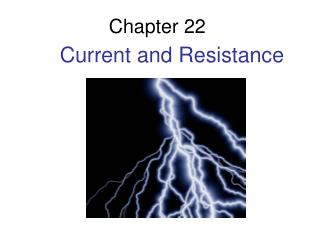Download PresentationChapter 22

# Chapter 22 - PowerPoint PPT Presentation

Chapter 22. Current and Resistance. Chapter 22 Current and Resistance. Topics:. Current Conservation of current Batteries Resistance and resistivity Simple circuits. Sample question:.I am the owner, or an agent authorized to act on behalf of the owner, of the copyrighted work described.
Download Presentation## Chapter 22

An Image/Link below is provided (as is) to download presentation

Download Policy: Content on the Website is provided to you AS IS for your information and personal use and may not be sold / licensed / shared on other websites without getting consent from its author.While downloading, if for some reason you are not able to download a presentation, the publisher may have deleted the file from their server.

- - - - - - - - - - - - - - - - - - - - - - - - - - E N D - - - - - - - - - - - - - - - - - - - - - - - - - -
Presentation Transcript
1. Chapter 22 Current and Resistance

2. Chapter 22 Current and Resistance Topics: • Current • Conservation of current • Batteries • Resistance and resistivity • Simple circuits Sample question: How can the measurement of an electric current passed through a person’s body allow a determination of the percentage body fat? Slide 22-1

3. How can we control the flow of electric charges? • Study the basic properties of current • Understand how our investigations of static electricity relates to current.

4. Charged Parallel Plate Capacitor • Connect the plates with a metal wire and the capacitor becomes discharged (neutral) • The capacitor is discharged by a current in the connecting wire.

5. Properties of a Current Indicators of Current in the Wire • Current is the motion of charges • Charges flow not current • Current is the flow Slide 22-8

6. Charge Carriers • Charge carriers are the charges that move in a current • The charge carriers in metals are electrons.

7. Conduction Electrons in a Metal

8. Charge Carriers • Insulator – does not have such free charges and cannot carry current • Semiconductor – intermediate case – few charge carriers which can be either positive or negative ions • Examples of ionic solutions as charge carriers – seawater, blood, intercellular fluids

9. Creating a Current • We use an electric field to “push” on the electrons in in a conductor similarly to pushing a book on a table to give it kinetic energy • When we apply an electric field the field exerts a force on the electrons and they begin to move.

10. Motion of electrons • Kinetic energy of collisions in converted to thermal energy • The metal gets warmer

11. Sustaining the current • You must continue the push or the electrons will stop moving • Maintain an electric field • Drift Velocity - Electron’s average motion is opposite the field • If the field goes to zero then the drift velocity goes to zero. • A current is a motion of charges sustained by an internal electric field

12. Where does the electric field come from in a current carrying wire? • A potential difference creates a current in the wire. • Electric potential energy Uelecis energy stored in a system of charged particles due to their electrical interactions. • The energy transfer Δ Uelec • The potential is measured in volts

13. Creating Potential • Electrons are bumped and pushed along • The E field has some strength in N/C • This is similar to the strength of a gravitational field in N/kg. (9.8N/kg) • Think about gravitational potential energy being mgh and electrical potential energy being qEd

14. Creating Potential • Uelec = qEd energy stored in a particle • Ug = mgh energy stored by height • E d = J/C =ΔV energy independent of charge • gh =J/kg • We care about the change in potential • Protons go from high to low potential • Electrons got from low to high potential

15. Creating Potential • In reality the electrons are moving • A battery adds energy • Think about an energy elevator. • A 1.5 V battery is like a charge escalator • The battery reenergizes the particle • The volt is the unit of potential • Voltage is the difference in potential • A particle uses all of its potential in a circuit

16. Simple Circuits The current is determined by the potential difference and the resistance of the wire: ∆Vchem R _____ I = Slide 22-13

17. Batteries The potential difference between the terminals of a battery, often called the terminal voltage, is the battery’s emf. Wchem q ____ ∆Vbat = = Slide 22-12

18. Difference in Potential creates current

19. Capacitor • The potential difference across the capacitor depends on the capacitor’s charge. • The potential difference (and the current) steadily falls as the capacitor discharges • When the capacitor is fully discharged there is no longer a potential difference and the current ceases

20. Add a lightbulb between two capacitors How does the current at A compare to current at B/ They are the same Conservation of Current

21. Conservation of Current • The number of electrons is not changed by the lightbulb • The lightbulb cannot destroy electrons • The lightbulb can’t store electrons • It does use energy

22. Law of Conservation of Current • The current is the same at all points in a current carrying wire

23. Definition of a Current Slide 22-9

24. Current • Current is a rate • The current direction in a wire is from higher potential to lower potential (in the direction of the electric field) • Unit is ampere • 1ampere = 1 coulomb per second = 1C/s

25. Conservation of Current Slide 22-10